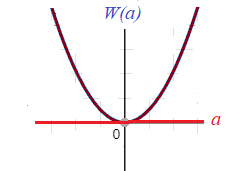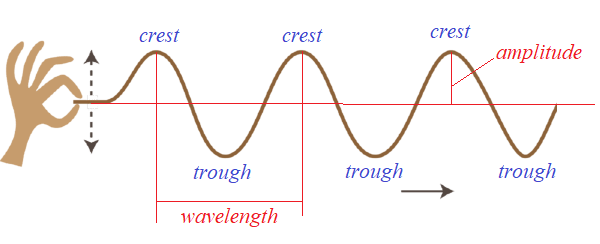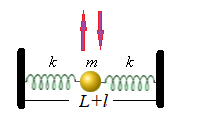## Sunday, October 17, 2021

### Musical Strings 2: UNIZOR.COM - Physics4Teens - Waves - Transversal Waves

Notes to a video lecture on http://www.unizor.com

Musical Strings 2

In the previous lecture we considered the model of a musical string as a point-mass m between two identical springs of the length (L+l)/2 each (where L is a neutral length of the string we are modeling and l is a stretch to create initial tension) with a coefficient of elasticity k.Plucking a string is, therefore, modeled as an initial displacement of a point-mass vertically by y(0)=a.

The result of the analysis of forces acting on a point-mass between these springs, when it performs transverse oscillations, was a differential equation
y"(t) + 2·(κ/m)·y(t)·
·
[1−L/(L+l)²+4y²(t)] = 0

Let's examine this model from the energy standpoint.
By lifting a point-mass initially by distance y(0)=a, we perform some work against elastic forces of two springs. The forces are variable and depend on how much we stretch the springs. Let's take coordinate y as our argument. Then, as explained in the previous lecture, the forces of two springs acting along the Y-axis against our motion total to
F↓↓(y) = −2·κ·y·
·
[1 − (L/2) /((L+l)/2)²+y²] =
= −2·κ·y·
[1 − L /(L+l)²+4y²]
Incidentally, it's easy to see that an expression in [...] is always positive, therefore the sign of the force is always opposite to the sign of a displacement y.

On an interval from y to y+dy of the infinitesimal length dy we can assume the constant force, as described above.
Therefore, the work we perform on that infinitesimal interval by going against the force F↓↓(y) is
dW = −F↓↓(y)·dy

We can now calculate the total work performed by displacing our point-mass by distance y(0)=a by integrating the above expression from y=0 to y=a.
W[0,a] = −[0,a]F↓↓(y)·dy =
=
[0,a]2·κ·y·
·
[1−(L/2) /((L+l)/2)²+y² ]·dy =
=
[0,a]2·κ·y·
·
[1−L/(L+l)²+4y² ]·dy

This integral can be calculated.
W[0,a] = 2·κ·[y²/2 −
− (L/4)·√(L+l)²+4y²
] |[0,a] =
= 2·κ·
[a²/2 −
− (L/4)·√(L+l)²+4a² +
+ L·(L+l)/4
] =
= κ·
[a² − (L/2)·√(L+l)²+4a² +
+ L·(L+l)/2
]

Just to check, if a=0, the work performed is
W[0,0] =
= κ·
[0² − (L/2)·√(L+l)²+0² +
+ L·(L+l)/2
] = 0
as it is supposed to be.

Analysis of the potential energy W(a) as a function of the initial displacement a shows that it resembles the parabola with a graph like this:So, potential energy is always positive, as it should be, and grows with initial displacement a growing to positive or negative direction (up or down on a picture of a point-mass between two springs above).

At any displacement point y from the neutral position a point-mass m has certain potential and kinetic energy.
Its potential energy W(y), as a function of displacement y, looks exactly the same as the one we calculated at the initial position, when y=a, which yields
W(y) =
= κ·
[y² − (L/2)·√(L+l)²+4y² +
+ L·(L+l)/2
]

Obviously, this formula is valid only for −a ≤ y ≤ a.
The difference between W[0,a] and W(y) is the kinetic energy our point-mass possesses because it moves towards the neutral position, that is
K(y)=m·(y')²/2.

Therefore, we have an equation, which is a differential equation for a displacement y(t) as a function of time t (in many cases we will drop (t) for brevity).
W(y) + K(y) = W[0,a]

The differential equation for displacement y(t) is
κ·[y² − (L/2)·√L+l)²+4y² +
+ L·(L+l)/2
] + m·(y')²/2 =
= κ·
[a² − (L/2)·√(L+l)²+4a² +
+ L·(L+l)/2
]

Simplifying...
y² − (L/2)·√(L+l)²+4y² +
+ (m/κ)·(y')²/2 =
= a² − (L/2)·√(L+l)²+4a²

or
(m/2κ)·(y')² + y² −
− (L/2)·√(L+l)² + 4y² =
= a² − (L/2)·√(L+l)²+4a²

This is a different differential equation that describes the transverse oscillations of a point-mass between two springs than that described in the previous lecture.
It contains only the first derivative of a displacement y(t).

Interestingly, though not surprisingly, this equation is not really different.
If two functions are equal, their derivatives are also equal. Take a derivative from both sides of an equation above:
(m/2κ)·2y'·y" + 2y·y' −
− (L/2)·8y·y' /2√(L+l)² + 4y² =
= 0

or
(m/2κ)·y'·y" + y·y' −
− L·y·y' /(L+l)² + 4y² =
= 0

Now we can cancel y'(t) getting
(m/2κ)·y" + y −
− L·y /(L+l)² + 4y² = 0

or
(m/2κ)·y" + y·
·
[1 − L /(L+l)² + 4y²] = 0
or
y" + (2κ/m)·y·
·
[1 − L /(L+l)² + 4y²] = 0
which is exactly the same as the equation derived in the previous lecture.

## Friday, October 8, 2021

### Musical Strings 1: UNIZOR.COM - Physics4Teens - Waves - Transversal Waves

Notes to a video lecture on http://www.unizor.com

Musical Strings 1

Our first example of transverse oscillations was the one with a rope, one end of which we shake up and down, with another end free.(open this picture in a new tab of your browser by clicking the right button of a mouse to better see details)

Now we will consider another type of transverse oscillations that is used in string musical instruments, like a guitar or violin.

The real oscillations of a violin string are quite complex, so we will have to rely on some simplified model that allows analytical approach.
So, here is the first really simple model of an oscillating string.

String of a violin or a guitar has two ends fixed and it oscillates when it's plucked.
The mechanism of plucking involves stretching a string at some middle point perpendicularly to a string direction and letting it go.

It is important to notice that real guitar or violin strings are initially stretched to create some tension, without which there will be no pleasant sound.
Also important is that real strings have a high coefficient of elasticity, so even a small stretch creates significant tension force.

In our simplified model we replace a string with two identical weightless springs of combined length L in a neutral (not stretched, nor squeezed) state, but stretched by a combined increment l to create initial tension, and a point-mass m fixed in the middle between the springs.
So, the neutral length of each spring is L/2 and the length increment of each spring, as it is initially stretched, is l/2.According to the Hooke's Law, the tension caused by initial stretch of each spring is
T = k·l/2
The initial tension forces of both springs act on a point-mass in the middle with equal magnitude and opposite directions and neutralize each other.

Plucking the point-mass in the upward direction, thus further stretching both springs it's attached to, and letting it go resembles the plucking of a string on a musical instrument, like a guitar or a violin.
The point-mass will start oscillating in the vertical direction, performing transversal oscillations.

This simplified model allows for analytical approach to find the differential equation of this type of oscillation.
Let's state in advance that the differential equation obtained will not yield to an easy solution, so we will just explain how to get to it, but then switch to another way to approach this problem.

Let's examine the forces acting on our point-mass after we lift it by the initial distance a and let it go, so it's performing vertical oscillations with y(t) being a deviation from the initial position at time t.

The initial condition of this motion is, therefore,
y(0) = a
y'(0) = 0

Assume that at time t the vertical deviation of a point-mass from its initial position is y(t). Then the two forces from two stretched springs acting on this point-mass are in red on a picture below.The forces can be evaluated using the Hooke's Law.
The neutral (unstretched) length of each spring is L/2, but it's initially stretched by l/2. The new stretched length is ((L+l)/2)²+y²(t). The force is proportional to elongation of a spring with a coefficient κ - spring's elasticity.
Therefore, each force by absolute value equals to
|F(t)| =
= κ·
[((L+l)/2)²+y²(t) − L/2]

We need to account only for vertical components of these two forces since horizontal components will act against each other and neutralize each other.
If each spring makes an angle θ with horizontal line, the absolute value of the vertical component of each force is
|F(t)| = |F(t)|·sin(θ)
In terms of y(t) the vertical component of both forces is
F(t) =
= −|F(t)|·y(t) /((L+l)/2)²+y²(t)

Since vertical components from both springs are added together, the total force F↓↓(t) acting on a point-mass is double the above expression, which should be simplified as follows.
F↓↓(t) = m·y"(t) =
= −2·|F(t)|·
·y(t) /(L+l/2)²+y²(t) =
= −2·κ·y(t)·
·
[1 − (L/2) /(L+l/2)²+y²(t)]

This gives us a differential equation of a transverse movement of a point-mass between two springs
m·y"(t) = −2·κ·y(t)·
·
[1 − (L/2) /((L+l)/2)²+y²(t)]

y"(t) + 2·(κ/m)·y(t)·
·
[1−(L/2)/((L+l)/2)²+y²(t)] = 0

If we consider the above equation as it is, it's too complex to get a nice solution. In cases like this physicists usually resort to reasonable approximations.

In this case we can safely assume that vertical deviation y(t) is small relatively to a the length L and, therefore, adds an insignificant amount to a radical in the denominator. So, let's just drop it from the equation, getting after a series of trivial steps a simpler differential equation
(a) y"(t) + 2·(κ/m)·y(t)·
·
[1−(L/2)/((L+l)/2)²] = 0
(b) y"(t) + 2·(κ/m)·y(t)·
·
[1−(L/2)/((L+l)/2)] = 0
(c) y"(t) + 2·(κ/m)·y(t)·
·
[1−L/(L+l)] = 0
(d) y"(t) + 2·(κ/m)·y(t)·
·l /(L+l) = 0

(e) y"(t) + ω²·y(t) = 0
where
ω² = 2·κ/[m·(1+L/l)]

The final equation is a familiar differential equation that describes harmonic oscillations with angular frequency ω.

From the expression
ω² = 2·κ/[m·(1+L/l)]
we see that greater initial stretch of our springs l contributes to higher angular frequency of oscillations, which corresponds to our experience with real strings - more initial tension applied to a string results in a higher tone of vibrations.

Considering initial conditions
y(0) = a and y'(0) = 0,
the vertical displacement of our point-mass between two springs will be
y(t) = a·cos(ωt)
where ω is evaluated above.